# How to Count Cells that do not Contain Errors in Excel

This post will guide you how to count the number of cells that do not contain errors within a range of cells using a formula in Excel 2013/2016 or Excel office 365. How do I count the number of cells without any errors using a simple formula in Excel.

## Count Number of Cells that do Not Contain Errors

You can use the SUMPRODUCT function in combining with NOT and ISERROR functions to count cells in a specified range of cells that do not contain errors

The below is a generic formula to count the number of cells that do not contain errors:

=SUMPRODUCT(–NOT(ISERR(range)))

Note: range is the range in which you want to count cells with no errors in the above generic form of the formula.

Supposing that you wish to count the number of cells in range B1:B6 that do not contain errors, and you can use the following formula:

=SUMPRODUCT(–NOT(ISERR(B1:B6)))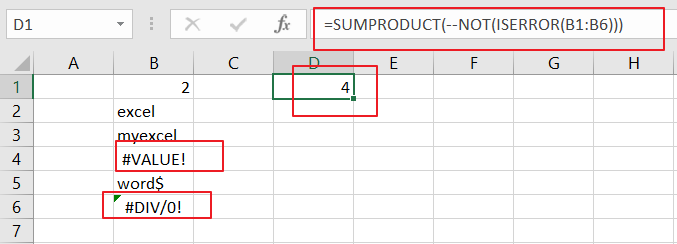LET’S SEE THAT HOW THIS FORMULA WORKS:

= NOT(ISERR(B1:B6))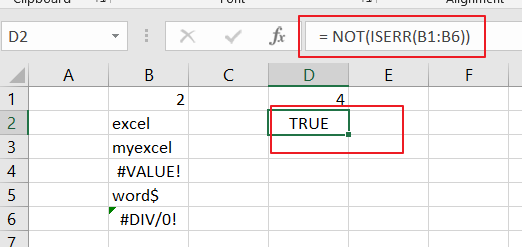The NOT and ISERROR functions can be used to identify the cells that do not contain an error. And it returns an array result with TRUE or FALSE values. The TRUE values indicate cells without errors, and FALSE values indicate cells with error values.

The array result is like this:

{TRUE;TRUE;TRUE;FALSE;TRUE;FALSE}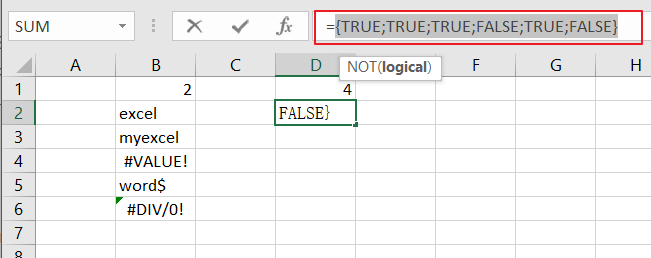= –NOT(ISERR(B1:B6))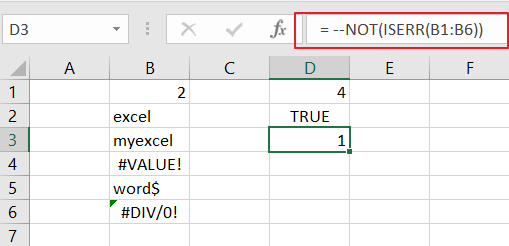Then you still need to convert the above array result to their numeric values, 1 and 0, so that the SUMPRODUCT function can calculate the sum of products of corresponding numbers. And you can do this with a double negative operator. And the final array result is like this:

{1;1;1;0;1;0}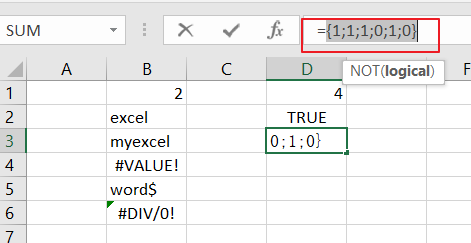The SUMPRODUCT function sums the items in the above array and return the final result.

You can also use the SUM function to count the cell that do not contain errors in range B1:B6. And the generic formula is similar with the above formula. It need to be entered as an array formula through pressing `CTRL +SHIFT + Enter` to convert it as array formula. The formula looks like this:

{=SUM(–NOT(ISERROR(B1:B6)))}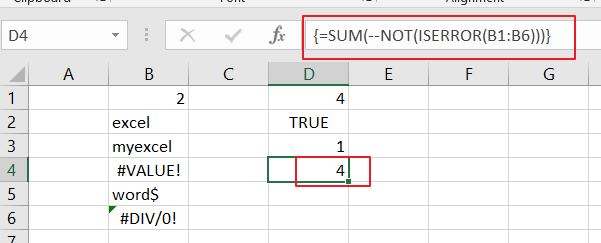### Related Functions

• Excel SUMPRODUCT function
The Excel SUMPRODUCT function multiplies corresponding components in the given one or more arrays or ranges, and returns the sum of those products. The syntax of the SUMPRODUCT function is as below:= SUMPRODUCT (array1,[array2],…)…
• Excel ISERROR function
The Excel ISERROR function used to check for any error type that excel generates and it returns TRUE for any error type, and the ISERR function also can be checked for error values except #N/A error, it returns TRUE while the error is #N/A. The syntax of the ISERROR function is as below:= ISERROR (value)….
• Excel SUM function
The Excel SUM function will adds all numbers in a range of cells and returns the sum of these values. You can add individual values, cell references or ranges in excel.The syntax of the SUM function is as below:= SUM(number1,[number2],…)…
• Excel NOT function
The Excel NOT function returns the opposite of a given logical or Boolean value. For example, if you supplied with the value TRUE, the NOT function will return FALSE; If you supplied with the value FALSE, and the NOT function will TRUE. The syntax of the NOT function is as below:=NOT(logical)…

Related Posts

Calculate Win Loss Tie

Suppose you got a task to calculate the win, loss, and tie totals; what would you do? If you are new to Ms Excel and don't have enough experience with it, then you might do this task manually but let ...

Count Cells that are Case Sensitive

If you are a frequent user of Microsoft Excel, you may have come across situations where you needed to add or count the cells that were case-sensitive. If you have done this task manually, I would like to point out ...

Check Dates in chronological order

Assume you have a date list that has different date formats, as seen in the accompanying picture. In this instance, Excel's Sort function will fail to sort them appropriately. However, you may convert all various date formats to a particular ...

Case Sensitive Lookup with SUMPRODUCT and EXACT

Today, we will show you how to use SUMPRODUCT and EXACT to perform a case sensitive exact match. In this article, we provide a simple example to calculate bonus for employees whose names are case-sensitive. If you meet similar scenarios ...

Average per Week by Formula in Excel

We usually apply AVERAGE function or relevant functions to return average directly in Excel worksheet. But in some situations, only applying average relevant functions cannot figure out our problem. Sometimes we can create a formula with functions and mathematical operation ...

How to Sum if Equal to Many Items or A Range in Excel

If we want to sum numbers from a range with criteria, we often select SUMIF of SUMIFS functions as the first choice to create a formula. The criteria can be a number or an array or a collection of some ...

How to Sum if Equal to X or Y in Excel

In daily work, if we want to sum numbers from a range, and only sum the numbers which being equal to X or Y in the range, we can create a formula with Excel build-in functions to get the result. ...

How To Sum the Largest N Values in Excel

Sometimes we may want to sum the largest N numbers or top N numbers in a range. In this article, we will show you the method of “SUM the Largest N Numbers” by a simple formula which consist of SUMPRODUCT ...

How to Sum for Cell Contains Formula Only in Excel

Sometimes values are created by formulas in cells. If we want to sum values which are created by formulas from a range, but some values which are hardcoded also list in the same range, how can we filter out matched ...

How to Sum the Smallest N Values in Excel

Sometimes we may want to sum the first smallest N numbers in a range in Excel. In this article, we will show you the method of “SUM the Smallest N Numbers” by a simple formula which consist of SUMPRODUCT and ...

Sidebar